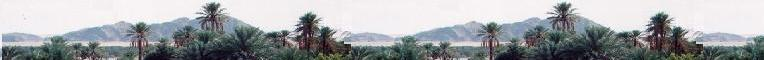Calculus II

Contents

Series

Definite integrals

Some primitives

Numerical methods

Exercices

# Calculus II: The criteria of convergence of series

It is often difficult to find the general term of a series. Often, we need just to know whether a series is convergent or divergent. In this case we use the criteria.

### 1. The divergence criterion

1.1. The rules are:

a) Rule 1:

If the series Σ1 an converges, then the related sequence {an} converges,
that is :

lim an = 0
n → + ∞

b) Rule 2:

The contrapositive (converse) proposition is of course true.

If the sequence {an} diverges, then the associated series Σ1 an diverges.
Or

If

lim an ≠ 0
n → + ∞
Then the related series Σ1 an diverges.

c) Rule 3:

But the reciprocal proposition is not true. That is:

If the sequence {an} converges, the associated series Σ1 an is not convergent.

Or

If

lim an = 0
n → + ∞
the associated series Σ1 an is not convergent.

1.2. Examples:

b) Rule 1:

The series Σ1 (n/(n + 1)) converges, then the related sequence {1/n(n + 1)} converges, that is :

lim (1/n(n + 1)) = 0
n → + ∞

b) Rule 2:

lim (n(n + 1)/2) ≠ 0
n → + ∞
Then the related series Σ1 (n(n + 1)/2) diverges.

c) Rule 3:

To be convergent, the codition an = 0 is necessary for the series Σ1 an, but not sufficient. That is the proposition:
1 an converges) implies
(lim an = 0
n → +∞),
but the converse is not true:
(lim an = 0
n → +∞)
does not imply (Σ1 an converges).

Here is an example, the harmonic series:
(lim (1/n) = 0
n → +∞)
and yet (Σ1(1/n) diverges.

### 2. D'Alembert criterion

The d'Alembert criterion or the ratio criterion is a test to define the convergence of a series.

The ratio test is written as:

Let the series: Σ1 an

L = lim |an+1/an|
n → + ∞

The d'Alembert's ratio states:

if L < 1 then the series converges,
if L > 1 then the series does not converge,
if L = 1 or the limit does not exists,
then the test is not conclusive.

There are convergent series for which L = 1 and divergent series for which L = 1. Therefore, when L = 1 or L fails to exist, test is inconclusive.

The d'Alembert's ratio test is efficient when the series for which the terms contain exponents "n" or n!.

#### Examples

a) Σ1 (n2n/n!)

 L = lim n → + ∞ |an+1/an|

an+1/an = (n+1) n! 2n+1/n (n + 1)!2n = 2/n

|an+1/an| = |2/n| = 2/n

 L = lim n → + ∞ 2/n = 0

L < 1, the series converges.

b) Σ1 ((- 1)n en2)

 L = lim n → + ∞ |an+1/an|

an+1/an = (- 1)n+1 e(n+1)2)/en2) =
(- 1) e(2n+1)

|an+1/an| = |(- 1) e(2n+1)| = e(2n+1)

 L = lim n → + ∞ e(2n+1) = + ∞
The series diverges.

c) The harmonic series Σ1 (1/n) diverges. The d'Alembert test is not efficient to conclude for this series. Indeed:

 L = lim n → + ∞ |an+1/an|

an+1/an = (1/(n + 1))/(1/n) = n/(n + 1)

|an+1/an| = n/(n + 1)
 L = lim n → + ∞ n/(n + 1) = n/n = 1
We cannot conclude.

### 3. Exercises

 chimie labs | Physics and Measurements | Probability & Statistics | Combinatorics - Probability | Chimie | Optics | contact |